# Top Books on Time Series Forecasting With R

Time series forecasting is a difficult problem.

Unlike classification and regression, time series data also adds a time dimension which imposes an ordering of observations. This turns rows into a sequence which requires careful and specific handling.

In this post, you will discover the top books for time series analysis and forecasting in R. These books will provide the resources that you need to get started working through your own time series predictive modeling problems.

The 5 top books covered in this post include:

1. Introductory Time Series with R.
2. Time Series Analysis and Its Applications: With R Examples.
3. Time Series Analysis: With Applications in R.
4. Multivariate Time Series Analysis: With R and Financial Applications.
5. Practical Time Series Forecasting with R: A Hands-On Guide.

Is your favorite time series in R book missing from this list?
Let me know in the comments.

Let’s dive in.

## Time Series Topics To Look Out For

Before we begin, a good book on time series depends on what you are looking for.

I don’t know any books really focus on machine learning for time series per se. Instead, books on time series analysis and forecasting focus on covering a suite of classical methods, such as:

• Regression Models.
• ARIMA models.
• Spectral analysis models.
• State-space models.

Books may also cover more modern techniques, such as:

• Resampling techniques.
• Categorical time series analysis.
• Multivariate spectral methods.
• Long memory Models.
• Nonlinear models
• GARCH models.
• ARMAX models.

These are good topics to look out for when picking your ideal book on time series forecasting.

## 1. Introductory Time Series with R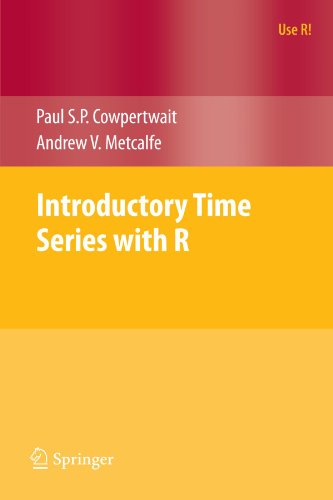Introductory Time Series with R assumes that you have completed a first-year statistics course.

A tutorial-based approach is used that focuses on a given time series prediction problem broken down into the following sections:

1. Motivation for the problem.
2. Model description.
3. Model demonstration on synthetic data.
4. Model is fitted to historical case study data.

The mathematics of the models is covered but can be skipped in favor on focusing on how to apply the models.

1. Time Series Data
2. Correlation
3. Forecasting Strategies
4. Basic Stochastic Models
5. Regression
6. Stationary Models
7. Non-stationary Models
8. Long-Memory Processes
9. Spectral Analysis
10. System Identification
11. Multivariate Models
12. State Space Models

Datasets, errata and source code for the book can be downloaded from the book’s homepage.

Below is a snippet from an Amazon review of the book:

This is an excellent introduction to time series analysis in R, and is suitable for all readers who use R. In contrast to most statistics books, it does not presume an extensive mathematical background. Rather, it is a very much a progressive, didactic text, suitable for leisurely self-learning. The mathematics are presented briefly and appropriately for each topic, but progress and understanding do not depend on absorbing them in depth. It would be suitable, for instance, to social scientists, ecologists, public policy researchers, and so forth who use R.

— Sitting in Seattle

## 2. Time Series Analysis and Its Applications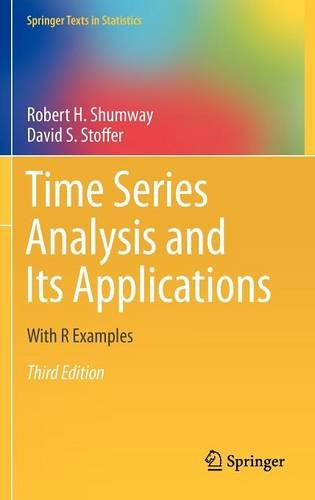Time Series Analysis and Its Applications: With R Examples is intended to be a textbook for undergraduate and graduate courses on time series.It assumes some background on regression analysis, and some mathematical statistics background.

It assumes some background on regression analysis, and some experience with mathematical statistics.

The book is structured so that theory and application are separated, allowing the book to be useful for different audiences, such as undergraduate and graduate students.

It is a popular book and has been through 4 editions.

1. Characteristics of Time Series
2. Time Series Regression and Exploratory Data Analysis
3. ARIMA Models
4. Spectral Analysis and Filtering
6. State-Space Models
7. Statistical Methods in the Frequency Domain

The supplementary information for the book can be downloaded from the book’s webpage.

Below is a snippet of a review of this book from Amazon.

This book covers all of the main areas of time series analysis such as ARIMA, GARCH and ARMAX models and spectral analysis and it does a pretty good job of it. Most of the explanations are clear enough for a beginner (with some statistical background) and are accompanied by worked examples (something which seems to be omitted in a lot of time series texts).

— Genevieve Hayes

## 3. Time Series Analysis: With Applications in R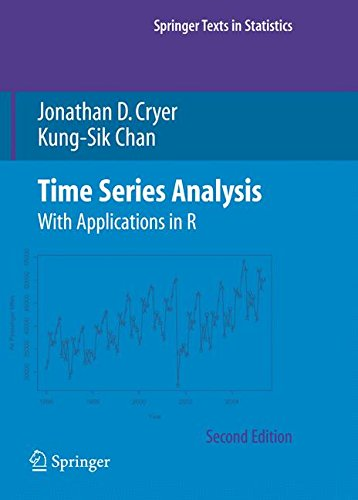Time Series Analysis: With Applications in R aims to cover both the theory of time series models and their application in R.

The book is intended for use as a one-semester university course and assumes a background in applied statistics with a focus on multiple linear regression and some calculus. Although some statistical concepts are reviewed in the Appendix.

1. Introduction
2. Fundamental Concepts
3. Trends
4. Models for Stationary Time Series
5. Models for Nonstationary Time Series
6. Model Specification
7. Parameter Estimation
8. Model Diagnostics
9. Forecasting
10. Seasonal Models
11. Time Series Regression Models
12. Time Series Models of Heteroscedasticity
13. Introduction to Spectral Analysis
14. Estimating the Spectrum
15. Threshold Models

The source code and datasets used in the book can be downloaded from the book’s website.

Below is a snippet from a review from Amazon.

Very well written, easy to understand. But it is a basic/elementary textbook. If I were learning time series on my own and wanted to use the R language, I would read this book first

— J. Gangolly

## 4. Multivariate Time Series Analysis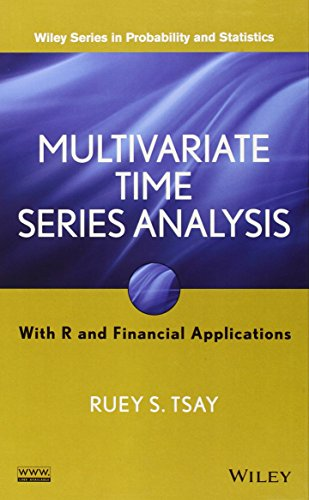Multivariate Time Series Analysis: With R and Financial Applications is based on the author’s 30 years teaching and researching multivariate time series analysis.

The book is intended to be used as a reference in graduate-level courses on time series and undergraduate statistics courses on time series. It does assume familiarity with univariate time series.

1. Multivariate Linear Time Series
2. Stationary Vector Autoregressive Time Series
3. Vector Autoregressive Moving-Average Time Series
4. Structural Specification of VARMA Models
5. Unit-Root Nonstationary Processes
6. Factor Models and Selected Topics
7. Multivariate Volatility Models

The functions and data used in the book are available in the MST R package. Source code and data can be downloaded from the book’s home page.

Below is a snippet from an Amazon review for the book.

The order by which topics are presented is reasonable. I like that the author starts right off with the more general vector/matrix approach and does not waste time introducing the “special case” posed by univariate time series, since this book is going to be very difficult for a student who has not already been exposed to the univariate case. Indeed, a level of familiarity with advanced mathematical topics (such as infinite-order matrix polynomials) is presumed. I also liked that proofs occur mostly at the end of chapters, and that the author maintains a web page with a listing of errata.

— Mindy

## 5. Practical Time Series Forecasting with R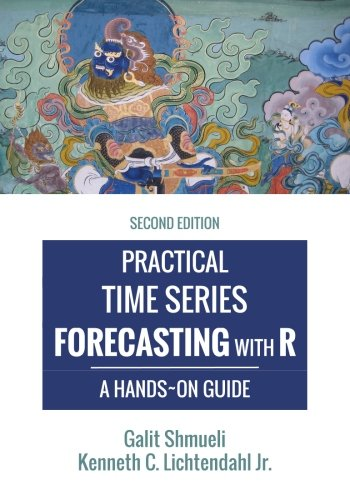Practical Time Series Forecasting with R: A Hands-On Guide is focused on a hands-on approach to teaching quantitative forecasting of time series.

The book was designed for use as a semester-long undergraduate or graduate course on time series forecasting.

1. Approaching Forecasting
2. Time Series Data
3. Performance Evaluation
4. Forecasting Methods: Overview
5. Smoothing Methods
6. Regression Models: Trends and Seasonality
7. Regression Models: Autocorrelation and External Info
8. Forecasting Binary Outcomes
9. Neural Networks
10. Communication and Maintenance
11. Cases

More information including datasets and source code can be found on the book’s homepage.

Below is a snippet from an Amazon review:

Really hits the sweet spot for someone looking for a gentle introduction to time series modeling: it covers all the bases (smoothers, ARIMA etc.), compares the pros and cons of different methods, discusses validation and testing, includes example code so you can get started right away and has just enough mathematical notation to simplify the descriptions of the methods. It’s succinct but not dumbed down. For how much it costs, you really can’t go wrong here.

— Stijn Debrouwere

## Summary

In this post, you discovered 5 top books on time series analysis and forecasting with R.

You now have the resources to bring the best predictive methods to your own time series predictive modeling problem.

### My Picks

For me personally, I picked up these two books:

• Introductory Time Series with R
• Practical Time Series Forecasting with R: A Hands-On Guide

Which books did you pick?

Have you read one of the books shared in this post?